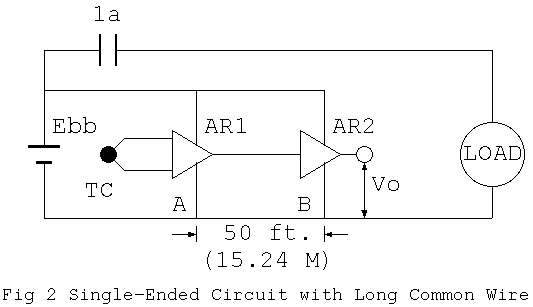# The Calculation of Inductance in IEEE Std 518-1977

The simplest case of electromagnetic interference (noise) is the one caused by common impedance coupling. There is a comment "IEEE Std 518-1977: IEEE Guide for the Installation of Electrical Equipment to Minimize Electrical Noise Inputs to Controllers from External Sources," as follows:The title of the Figure 2 means "unbalanced circuit sharing a long wire," where "TC" is a thermocouple, "AR1" and "AR2" amplifiers, and "1a" a contact to turn on and off "load" by using power source "Ebb." An electric wire of 50 ft long and of 12 AWG conductor size is used to connect "A" and "B," and is also commonly used by the thermocouple amplifier and the loaded control circuit which is controlled by the mechanical contact.

There are two subjects that this standard argues in this example, one interference of direct current and the other interference of transient phenomena.

Regarding the interference of direct current, the induced electromotive force between "A" and "B" by loaded current is 0.040 V, if the loaded current is 0.5 A, because the direct current resistance of the electric cable between "A" and "B" is 0.08 Ohm. Then, provided that the amplification ratio of "AR2" is 100, the error is, it says, approximately 4'F around 100'F of iron-constantan thermocouple. This must be easily confirmed.

Regarding the interference of transient phenomena, they consider to open the contact "1a" that has been closed. As the current changes from 0.5A to 0 in 1uS, self-inductance must be considered in case of mechanical contact. Therefore, the following formula:

```  L = 0.002*l*(log(2*l/r)-3/4)  (uH)

where,
L = self-inductance of a straight conductor
l = length of wire (cm)
r = radius of conductor (cm)
```
is presented as "self-inductance of a straight wire at high frequency." Under these conditions, l = 1524 cm and r = 0.205 cm and, therefore,
```  L = 3.05 * log((2*1524/0.205) - 3/4) = 27 uH
```
Furthermore, considering the following circuit characteristic of inductance:
```  Vab = L * (di/dt),
```
it is concluded that the following voltage is generated,
```  Vab = 27e- 6*(0.5/1e- 6) = 13.5 Vpeak
```
Regarding this formula of inductance, they say "normally inductance is a property attached to a complete circuit, but it is possible to attach a value of inductance to each segment making up a circuit. By this method, it is possible to calculate the induced electromotive force on the basis of time variation of the current," and conclude that the formula has been drawn from the following document,
```  Grover, F. W. - Inductance Calculations
New York: Van Nostrand, 1944, p35, Equation (7)
```

The "IEEE Stds," are the bases of electric and electronic technology of the world. Nonetheless, is the above calculation of "Vab" really reliable? For example, if the length of the wire is continued to be shortened, you may get negative figures eventually, which sounds strange.